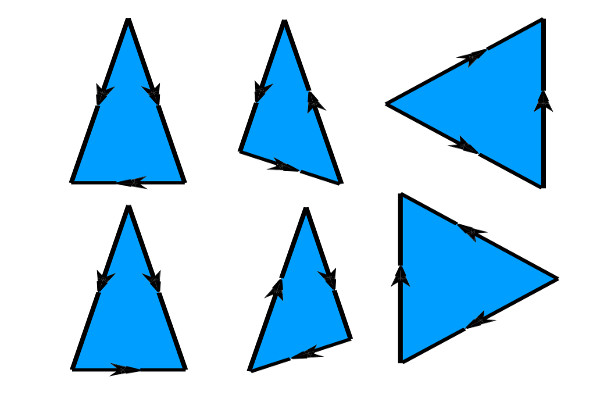## Challenge 4 - Help Pythagoras JuniorPythagoras Junior is not a great mathematician like his great great great grandfather. When he gets a list of numbers, he finds it hard to match 3 numbers and create a triangle out of them.

You need to help Pythagoras Junior to identify which is the smallest possible triangle given a list of side lengths. We define the smallest triangle as the one with the minimum perimeter.

### Input

The first line will contain an integer C, the number of cases for our problem.
Each case consists of a line starting with an integer N, the number of sides, followed by N integers, each indicating the length of a side. All the integers are separated by spaces.

### Output

For each case, a line starting with "Case #x: " followed by the perimeter of the smallest triangle or, if it's not possible to form a valid triangle, "IMPOSSIBLE". Every line is followed by a new line character.

### Examples

 ```Case 1: 6 13 9 1 13 17 6 ``` ```Case 2: 7 110 40 10 1 20 60 3 ```

In Case 1, the answer is 27, as the triangle with the smallest perimeter is (13, 13, 1).
In Case 2, the answer is IMPOSSIBLE. You cannot create a valid triangle from any three of the given numbers.

### Limits

• 3 ≤ N ≤ 105
• 1 ≤ Lengths ≤ 232

### Sample Input

```2
6 13 9 1 13 17 6
7 110 40 10 1 20 60 3```

### Sample Output

```Case #1: 27
Case #2: IMPOSSIBLE```